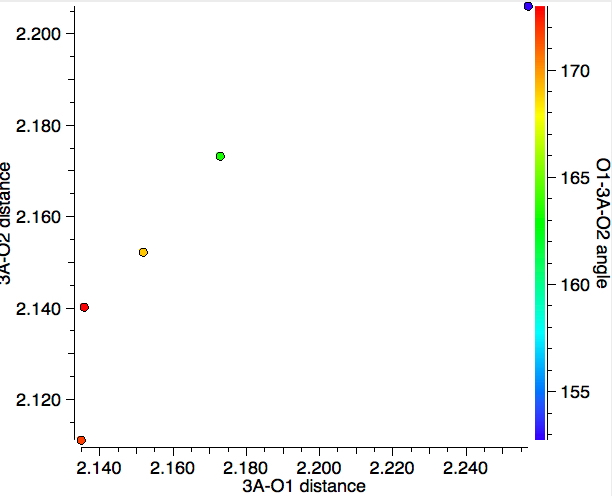## The geometries of 5-coordinate compounds of group 14 elements.

This is a follow-up to one aspect of the previous two posts dealing with nucleophilic substitution reactions at silicon. Here I look at the geometries of 5-coordinate compounds containing as a central atom 4A = Si, Ge, Sn, Pb and of the specific formula C34AO2 with a trigonal bipyramidal geometry. This search arose because of a casual comment I made in the earlier post regarding possible cooperative effects between the two axial ligands (the ones with an angle of ~180 degrees subtended at silicon). Perhaps the geometries might expand upon this comment?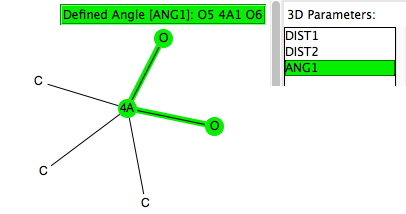The search query is shown above results in 394 hits (May 2016) and is presented with the three variables in the query plotted as below, with the O-4A-O angle indicated by colour (red ~ 180°; blue ~90° and green ~120°).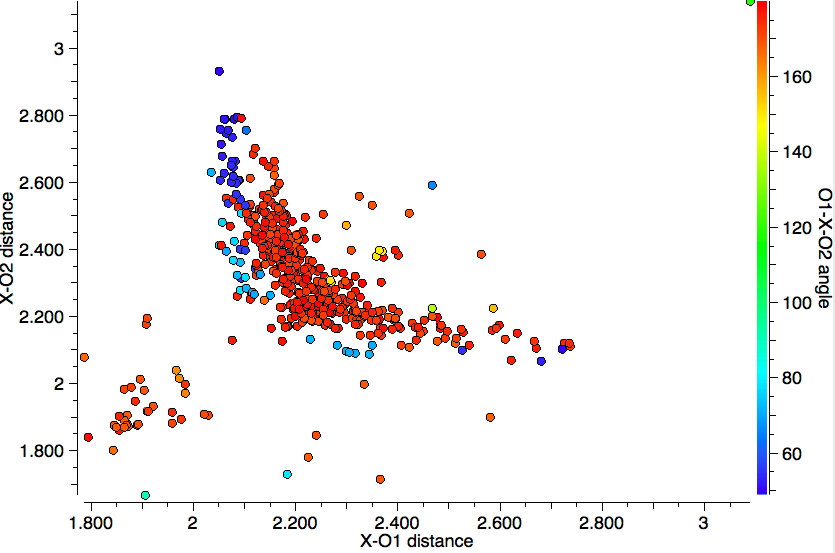1. The cluster at distances of 4A-O of ~1.9Å represents silicon compounds, and tends to suggest that the pair of distances 4A-O are quite similar in value. The angles correspond to a di-axial arrangement around the silicon. In this scenario, one might imagine a stereoelectronic effect similar to the anomeric effect when 4A = C operates and which has the potential to strengthen both di-axial oxygens.
2. The bulk of the points come at higher 4A-O distances of > 2.1Å and consist mostly of 4A = Sn. There are two a clear-cut distributions, one for angles of ~180° and a separate one for angles of ~90° and both are qualitatively different from the Si distribution. The 180° set corresponds to a di-axial arrangement for the oxygens, whereas the 90° set suggests an axial-equatorial geometry. Both distributions have prominent tails which reveal that as one 4A-O distance shortens, the other lengthens, equivalent to asymmetric anomeric effects at  O-C-O.
3. Noticeably absent are any green points; these would correspond to bond angles of ~120° and hence would correspond to di-equatorial ligands.

This quick exploration (with potential variations that I have not explored above) can be added to the collection of "ten minute explorations" I have described elsewhere.

## References

1. H.S. Rzepa, "Discovering More Chemical Concepts from 3D Chemical Information Searches of Crystal Structure Databases", Journal of Chemical Education, vol. 93, pp. 550-554, 2015. http://dx.doi.org/10.1021/acs.jchemed.5b00346

### 3 Responses to “The geometries of 5-coordinate compounds of group 14 elements.”

1.Henry Rzepa says:

Here I show three plots for the equivalent search for group 15 elements (5A). The first includes all group 15 elements, and the next two are specifically for X=P and X = Sb.

Note again the difference between X=P and the rest of the elements in this column.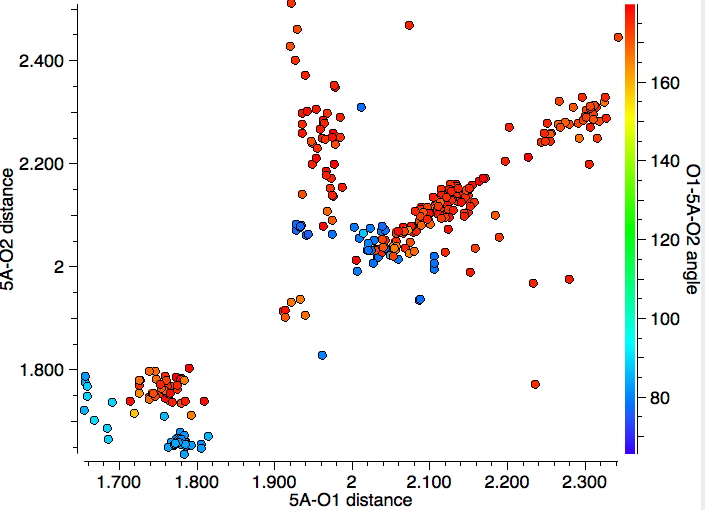The next plot is an expansion of the bottom left corner to restrict the search just to X=P. Note that for the cluster in the bottom right, we have an angle of ~90° with one axial and one equatorial oxygen, and revealing the difference in the P-O bond lengths for these two positions. In contrast, the cluster for di-axial oxygens seems to show no differences in the two P-O bond lengths.The next plot is for X=Sb, and reveals different behaviour compared to X=P. One cluster appears to show variation in which both Sb-O lengths increase in concert, and another cluster where one length varies whilst the other does not.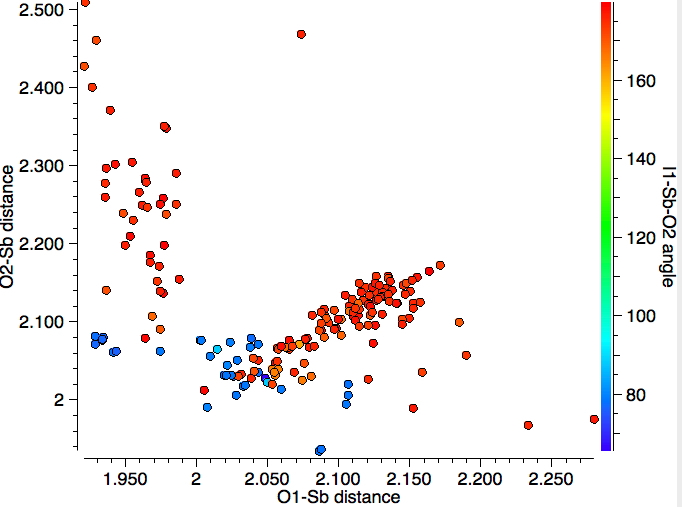2.Henry Rzepa says:

Here is a 1-off observation for 5-coordinate compounds of the type R36AO2, where the central atom is from group 16, and X=S. Now, the two oxygen atoms both occupy equatorial positions, allowing the (apparently) more electronegative CF3 groups to occupy the axial locations. See doi: 10.5517/CC6W6X5

3.Henry Rzepa says:

Here is the (much sparser) plot for group 13 elements (3A). About all one can conclude is that again the two electronegative oxygen atoms are di-axial.